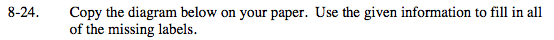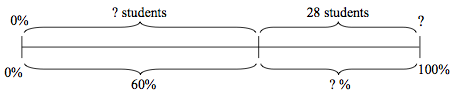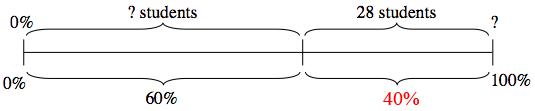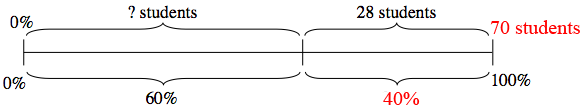### Home > MC2 > Chapter 8 > Lesson 8.1.2 > Problem8-24

8-24.First solve for the lower half of the diagram.

Since the entire diagram is representative of 100% of the students, and the first portion represents 60%, the second portion must represent 40%.28 students is 40% of how many total students?

To find the remaining amount of students for the first portion, subtract 28 from the total amount of students.

$\frac{40}{100}\left(\frac{?}{?}\right)=\frac{28}{x}$